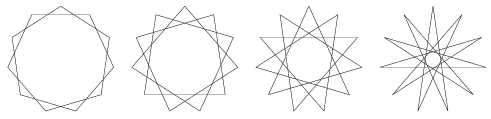Mathematical and Physical Journal
for High Schools
Issued by the MATFUND Foundation
 Already signed up? New to KöMaL?# Exercises and problems in InformaticsOctober 2003

I. 58. A sequence a1, a2, ..., an of positive integers each having at most N digits is called an aliquot sequence of N digits, if the sum of positive proper divisors (i.e. including 1 but excluding the number itself) of ai is ai+1 (i=1,2,...,n-1) and that of an is a1. Members of an aliquot sequence are called sociable numbers. (Thus, the aliquot sequences of length 1 are just the perfect numbers, and aliquot sequences of length 2 are the usual amicable pairs.)

Your program (i58.pas, ...) should read the value of N (1$\displaystyle \le$N $\displaystyle \le$8), compute every sociable numbers of N digits for which the smallest element is in the interval [A,B], then write the output into the text file i58.out''.

The file containing the output corresponding to parameter values N=7, A=2, B=9 999 999 should be submitted.

Examples. Sociable numbers of 3 digits with the smallest element in the interval [200,230] form the well-known amicable pair 220-284.

Sociable numbers of 5 digits with the smallest element in the interval [10000,13000] include 10744-10856, 12285-14595, 12496-14288-15472-14536-14264.

(10 points)

I. 59. A regular star polygon with N vertices is obtained by connecting every vertex of a regular N-gon with both of its Kth neighbours.

Write a program (i59.pas, ...) which reads the value of N (5$\displaystyle \le$N $\displaystyle \le$100), then displays all distinct regular star polygons with N vertices.

The example shows all 4 distinct regular star polygons with 11 vertices.

(10 points)I. 60. Similarly to the concept of highly composite numbers (see Problem I. 55. in the September 2003 issue), we say that a positive integer n $\displaystyle \in$[A,B] is highly composite with respect to the interval [A,B], if the number of divisors of n is greater than or equal to that of any positive integers in the interval [A,B] below n.

Prepare your sheet (i60.xls) which - if A and B (1$\displaystyle \le$A$\displaystyle \le$B$\displaystyle \le$1000) are given - displays all numbers in the interval [A,B] highlighting with red all highly composite numbers with respect to that interval.

In the example italic letters show the red highlighting.

 A=10 B=25 10 11 12 13 14 15 16 17 18 19 20 21 22 23 24 25

(10 points)